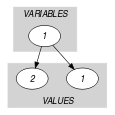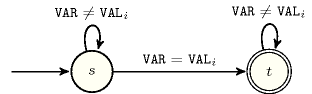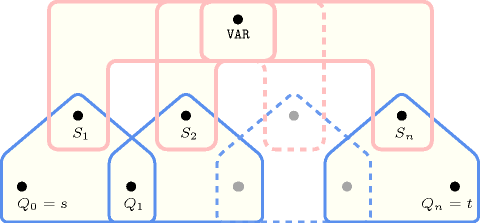## 5.177. in

Origin

Domain definition.

Constraint

$\mathrm{𝚒𝚗}\left(\mathrm{𝚅𝙰𝚁},\mathrm{𝚅𝙰𝙻𝚄𝙴𝚂}\right)$

Synonyms

$\mathrm{𝚍𝚘𝚖}$, $\mathrm{𝚒𝚗}_\mathrm{𝚜𝚎𝚝}$, $\mathrm{𝚖𝚎𝚖𝚋𝚎𝚛}$.

Arguments
 $\mathrm{𝚅𝙰𝚁}$ $\mathrm{𝚍𝚟𝚊𝚛}$ $\mathrm{𝚅𝙰𝙻𝚄𝙴𝚂}$ $\mathrm{𝚌𝚘𝚕𝚕𝚎𝚌𝚝𝚒𝚘𝚗}\left(\mathrm{𝚟𝚊𝚕}-\mathrm{𝚒𝚗𝚝}\right)$
Restrictions
 $|\mathrm{𝚅𝙰𝙻𝚄𝙴𝚂}|>0$ $\mathrm{𝚛𝚎𝚚𝚞𝚒𝚛𝚎𝚍}$$\left(\mathrm{𝚅𝙰𝙻𝚄𝙴𝚂},\mathrm{𝚟𝚊𝚕}\right)$ $\mathrm{𝚍𝚒𝚜𝚝𝚒𝚗𝚌𝚝}$$\left(\mathrm{𝚅𝙰𝙻𝚄𝙴𝚂},\mathrm{𝚟𝚊𝚕}\right)$
Purpose

Enforce the domain variable $\mathrm{𝚅𝙰𝚁}$ to take a value within the values described by the $\mathrm{𝚅𝙰𝙻𝚄𝙴𝚂}$ collection.

Example
$\left(3,〈1,3〉\right)$

The $\mathrm{𝚒𝚗}$ constraint holds since its first argument $\mathrm{𝚅𝙰𝚁}=3$ occurs within the collection of values $\mathrm{𝚅𝙰𝙻𝚄𝙴𝚂}=〈1,3〉$.

Typical
$|\mathrm{𝚅𝙰𝙻𝚄𝙴𝚂}|>1$
Symmetries
• Items of $\mathrm{𝚅𝙰𝙻𝚄𝙴𝚂}$ are permutable.

• $\mathrm{𝚅𝙰𝚁}$ can be set to any value of $\mathrm{𝚅𝙰𝙻𝚄𝙴𝚂}.\mathrm{𝚟𝚊𝚕}$.

• One and the same constant can be added to $\mathrm{𝚅𝙰𝚁}$ as well as to the $\mathrm{𝚟𝚊𝚕}$ attribute of all items of $\mathrm{𝚅𝙰𝙻𝚄𝙴𝚂}$.

Arg. properties

Extensible wrt. $\mathrm{𝚅𝙰𝙻𝚄𝙴𝚂}$.

Remark

Entailment occurs immediately after posting this constraint.

The $\mathrm{𝚒𝚗}$ constraint is called $\mathrm{𝚍𝚘𝚖}$ in Gecode (http://www.gecode.org/), and $\mathrm{𝚖𝚎𝚖𝚋𝚎𝚛}$ in MiniZinc (http://www.minizinc.org/). In MiniZinc the $\mathrm{𝚟𝚊𝚕}$ attribute is not necessarily fixed, i.e. it can be a domain variable.

Systems

member in Choco, rel in Gecode, dom in Gecode, in in JaCoP, member in MiniZinc, in in SICStus, in_set in SICStus.

Used in
Keywords
Derived Collection
$\mathrm{𝚌𝚘𝚕}\left(\mathrm{𝚅𝙰𝚁𝙸𝙰𝙱𝙻𝙴𝚂}-\mathrm{𝚌𝚘𝚕𝚕𝚎𝚌𝚝𝚒𝚘𝚗}\left(\mathrm{𝚟𝚊𝚛}-\mathrm{𝚍𝚟𝚊𝚛}\right),\left[\mathrm{𝚒𝚝𝚎𝚖}\left(\mathrm{𝚟𝚊𝚛}-\mathrm{𝚅𝙰𝚁}\right)\right]\right)$
Arc input(s)

$\mathrm{𝚅𝙰𝚁𝙸𝙰𝙱𝙻𝙴𝚂}$ $\mathrm{𝚅𝙰𝙻𝚄𝙴𝚂}$

Arc generator
$\mathrm{𝑃𝑅𝑂𝐷𝑈𝐶𝑇}$$↦\mathrm{𝚌𝚘𝚕𝚕𝚎𝚌𝚝𝚒𝚘𝚗}\left(\mathrm{𝚟𝚊𝚛𝚒𝚊𝚋𝚕𝚎𝚜},\mathrm{𝚟𝚊𝚕𝚞𝚎𝚜}\right)$

Arc arity
Arc constraint(s)
$\mathrm{𝚟𝚊𝚛𝚒𝚊𝚋𝚕𝚎𝚜}.\mathrm{𝚟𝚊𝚛}=\mathrm{𝚟𝚊𝚕𝚞𝚎𝚜}.\mathrm{𝚟𝚊𝚕}$
Graph property(ies)
$\mathrm{𝐍𝐀𝐑𝐂}$$=1$

Graph model

Parts (A) and (B) of Figure 5.177.1 respectively show the initial and final graph associated with the Example slot. Since we use the $\mathrm{𝐍𝐀𝐑𝐂}$ graph property, the unique arc of the final graph is stressed in bold.

##### Figure 5.177.1. Initial and final graph of the $\mathrm{𝚒𝚗}$ constraint(a) (b)
Signature

Since all the $\mathrm{𝚟𝚊𝚕}$ attributes of the $\mathrm{𝚅𝙰𝙻𝚄𝙴𝚂}$ collection are distinct and because of the arc constraint $\mathrm{𝚟𝚊𝚛𝚒𝚊𝚋𝚕𝚎𝚜}.\mathrm{𝚟𝚊𝚛}=\mathrm{𝚟𝚊𝚕𝚞𝚎𝚜}.\mathrm{𝚟𝚊𝚕}$ the final graph contains at most one arc. Therefore we can rewrite $\mathrm{𝐍𝐀𝐑𝐂}=1$ to $\mathrm{𝐍𝐀𝐑𝐂}\ge 1$ and simplify $\underline{\overline{\mathrm{𝐍𝐀𝐑𝐂}}}$ to $\overline{\mathrm{𝐍𝐀𝐑𝐂}}$.

Automaton

Figure 5.177.2 depicts the automaton associated with the $\mathrm{𝚒𝚗}$ constraint. Let ${\mathrm{𝚅𝙰𝙻}}_{i}$ be the $\mathrm{𝚟𝚊𝚕}$ attribute of the ${i}^{th}$ item of the $\mathrm{𝚅𝙰𝙻𝚄𝙴𝚂}$ collection. To each pair $\left(\mathrm{𝚅𝙰𝚁},{\mathrm{𝚅𝙰𝙻}}_{i}\right)$ corresponds a 0-1 signature variable ${S}_{i}$ as well as the following signature constraint: $\mathrm{𝚅𝙰𝚁}={\mathrm{𝚅𝙰𝙻}}_{i}⇔{S}_{i}$.

##### Figure 5.177.2. Automaton of the $\mathrm{𝚒𝚗}$ constraint##### Figure 5.177.3. Hypergraph of the reformulation corresponding to the automaton of the $\mathrm{𝚒𝚗}$ constraint